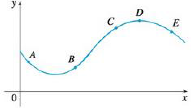Chapter 4.3, Problem 34E### Single Variable Calculus: Early Tr...

8th Edition
James Stewart
ISBN: 9781305270343

#### Solutions

Chapter
Section### Single Variable Calculus: Early Tr...

8th Edition
James Stewart
ISBN: 9781305270343
Textbook Problem

# The graph of a function y = f(x) is shown. At which point(s) are the following true?(a) d y d x and d 2 y d x 2 are   both   positive .(b) d y d x and d 2 y d x 2 are   both   negative .(c) d y d x is   negative   but d 2 y d x 2 is   positive .(a)

To determine

To find: The point which satisfy the mentioned condition.

Explanation

Given:

The condition is dydx and d2ydx2 both are positive.

Calculation:

From the given condition, it is observed that the first derivative of the function f(x) is positive. So, the function f(x) is increasing.

That is, since dydx>0 , f(x) is increasing

(b)

To determine

To find: The point which satisfy the mentioned condition.

(c)

To determine

To find: The point which satisfy the mentioned condition.

### Still sussing out bartleby?

Check out a sample textbook solution.

See a sample solution

#### The Solution to Your Study Problems

Bartleby provides explanations to thousands of textbook problems written by our experts, many with advanced degrees!

Get Started

#### Solve the equations in Exercises 126. 2x3x2x3x+1=0

Finite Mathematics and Applied Calculus (MindTap Course List)

#### Equations Solve the equation graphically. 142. |x+35|=2

Precalculus: Mathematics for Calculus (Standalone Book)

#### Does the point P (1, 54) lie on the line 6x 8y 16 = 0? Justify your answer.

Applied Calculus for the Managerial, Life, and Social Sciences: A Brief Approach

#### Sometimes, Always, or Never: If for all n and both {an} and {cn} converge, then{bn} converges.

Study Guide for Stewart's Single Variable Calculus: Early Transcendentals, 8th